# Tutored Practice Problem 16.4.3 couTS TOMARDS GRADI Calculate the pH of a weak acid solution (IHAlo>...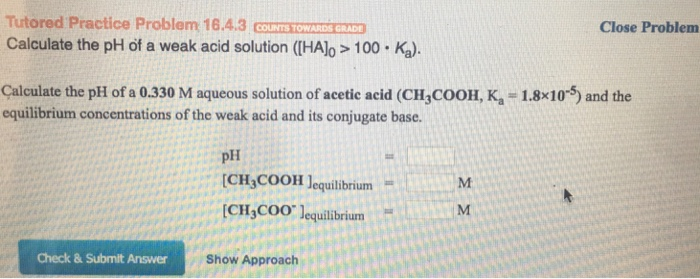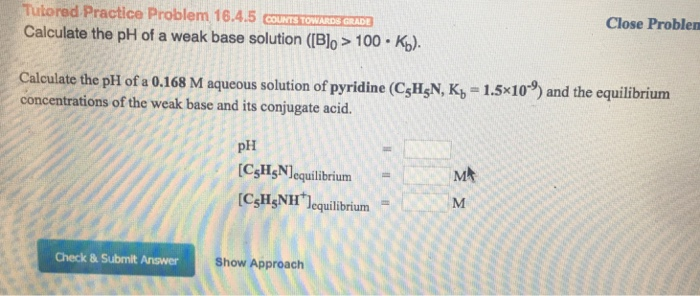Tutored Practice Problem 16.4.3 couTS TOMARDS GRADI Calculate the pH of a weak acid solution (IHAlo> 100 Ka). Close Problem Çalculate the pH of a 0.330 M aqueous solution of acetic acid (CH,COOH, K-1.8x105) and the equilibrium concentrations of the weak acid and its conjugate base. pH [CH3COOH ]equilibrium = I..Hmrt CH COO lequilibrium Check & Submit Answer Show Approach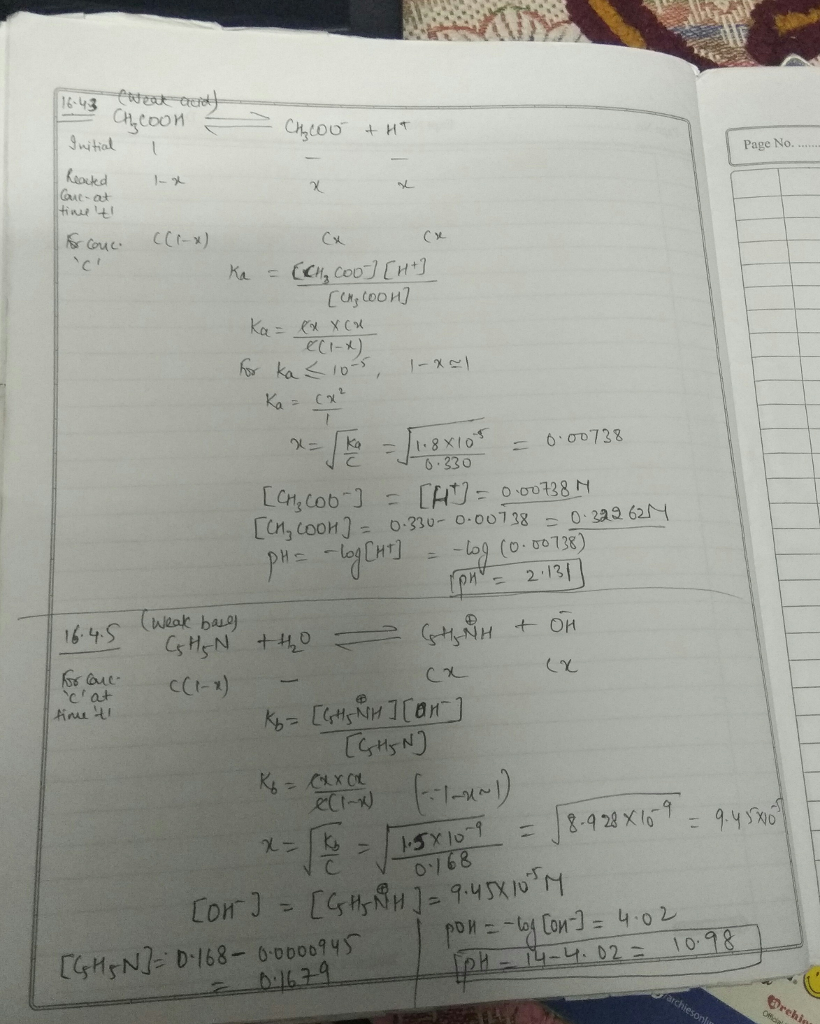#### Earn Coin

Coins can be redeemed for fabulous gifts.

Similar Homework Help Questions
• ### Calculate the pH of a weak acid solution Close Problem Tutored Practice Problem 16.4.3 STORE Calculate...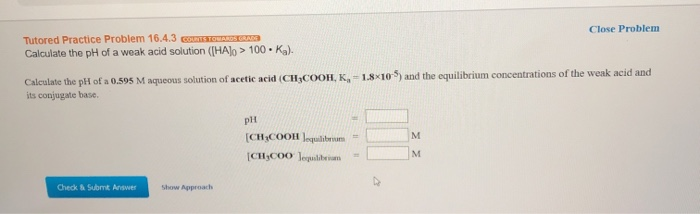Calculate the pH of a weak acid solution Close Problem Tutored Practice Problem 16.4.3 STORE Calculate the pH of a weak acid solution ([HAlo > 100. Ka). Calculate the pH of a 0.595 M aqueous solution of acetic acid (CH3COOH, K, -1.8*10) and the equilibrium concentrations of the weak acid and its conjugate base pH CHCOOH = CHC00 lm Check Submnt Awe Show Approach

• ### Calculate pH of a weak base solution. Please box answers! Thank you! Tutored Practice Problem 16.4.5...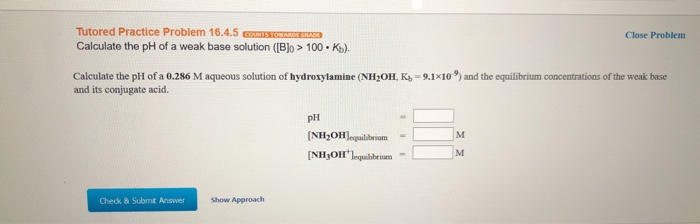Calculate pH of a weak base solution. Please box answers! Thank you! Tutored Practice Problem 16.4.5 G S GRASS Calculate the pH of a weak base solution ([B]o > 100Ko). Close Problem Calculate the pH of a 0.286 M aqueous solution of hydroxylamine (NH,OH, Kb - 9.1x10') and the equilibrium concentrations of the weak base and its conjugate acid. PH (NH3OH)equilibrium [NH3OH lequilibrium - Check & Submit Answer Show Approach

• ### Close Proble Tutored Practice Problem 16.4.3 COUNIS TOW Calculate the pH of a weak acid solution...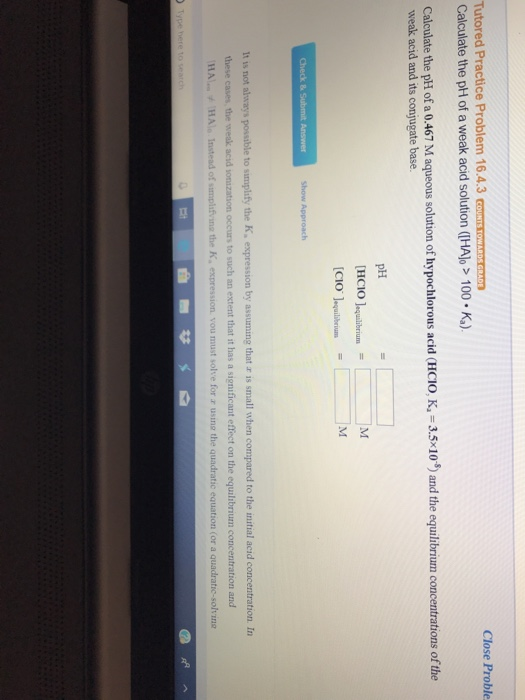Close Proble Tutored Practice Problem 16.4.3 COUNIS TOW Calculate the pH of a weak acid solution (HAJo >100 Ka) Calculate the pH of a 0.467 M aqueous solution of hypochlorous acid (HCIo, K, 3.5 10 weak acid and its conjugate base. and the equilbrium concentrations of the pH [FICIO!equilibrium CIoaquliborium Show Approach It is not always possible to simplify the K, expression by assuming that r is small when compared to the initial acid concentration. In these cases, the weak...

• ### Calculate pH of a weak base solution. Please box answers! Thank you! Close Problem Tutored Practice...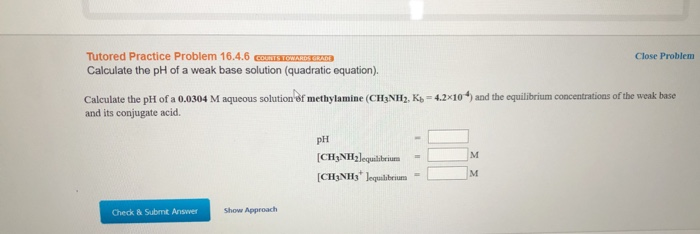Calculate pH of a weak base solution. Please box answers! Thank you! Close Problem Tutored Practice Problem 16.4.6 cm STOWARRANTIE Calculate the pH of a weak base solution (quadratic equation). Calculate the pH of a 0.0304 M aqueous solution of methylamine (CHANH. K. - 4.2x10*) and the equilibrium concentrations of the weak base and its conjugate acid PH [CH3NH2lequilibrium [CH3NH3 Jequilibrium - Check & Submnt Answer Show Approach

• ### Help plz Close Problens Calculate the pH of a weak acid solution (IHAb 100.Ka). Calculate the...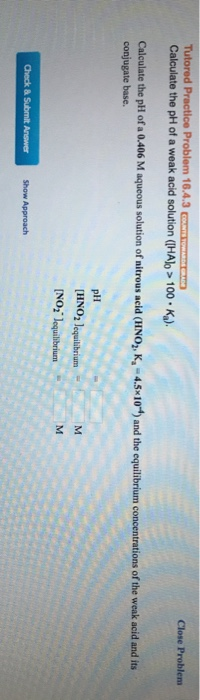Help plz Close Problens Calculate the pH of a weak acid solution (IHAb 100.Ka). Calculate the pH of a 0.406 M aqueous solution of nitrous acid (HNO,, K-4.5x10) and the equilibrium concentrations of the weak acid and its conjugate base. pHH [HNO2 lequilibrium NO2 lequilbrium Show Approach

• ### Calculate the pH of a weak acid solution using the quadratic formula. Please box answer! Thank...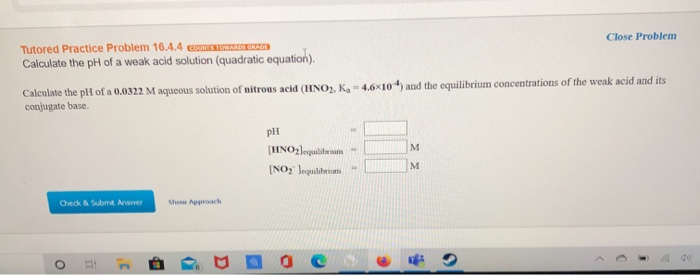Calculate the pH of a weak acid solution using the quadratic formula. Please box answer! Thank you! Close Problem Tutored Practice Problem 16.4.4 S O NDE Calculate the pH of a weak acid solution (quadratic equation). Calculate the pll of a 0.0322 M aqueous solution of nitrous acid (HINO), K-4.6x10") and the equilibrium concentrations of the weak acid and its conjugate base pH (HNO3Jequilibrium [NO2 loqulibrum Check Sem Arses Shopproach

• ### A)Calculate the pH of a weak base solution ([B]0 > 100 • Kb). Close Problem Calculate the pH o...

a)Calculate the pH of a weak base solution ([B]0 > 100 • Kb). Close Problem Calculate the pH of a 0.289 M aqueous solution of triethanolamine (C6H15O3N, Kb = 5.8×10-7) and the equilibrium concentrations of the weak base and its conjugate acid. pH = [C6H15O3N]equilibrium = M [C6H15O3NH+]equilibrium = M b) Calculate the pH of a 0.0338 M aqueous solution of dimethylamine ((CH3)2NH, Kb = 5.9×10-4) and the equilibrium concentrations of the weak base and its conjugate acid. pH =   ...

• ### Calculate the pH of a 0.408 M aqueous solution of nitrous acid (HNO,, K, -4.5x 10-4)...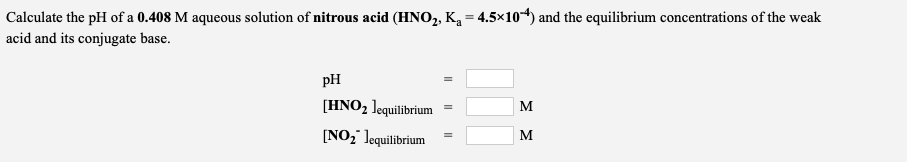Calculate the pH of a 0.408 M aqueous solution of nitrous acid (HNO,, K, -4.5x 10-4) and the equilibrium concentrations of the weak acid and its conjugate base. PH [HNO2 lequilibrium = [NO2 lequilibrium =

• ### Multi part question Tutored Practice Problem 17.2.3 COUNTS TOWARDS GRADE Calculate pH of a weak base/conjugate acid buffer solution A 0.420-M aqueous solution of C5H;N (pyridine) has a pH of 9.40. Ca...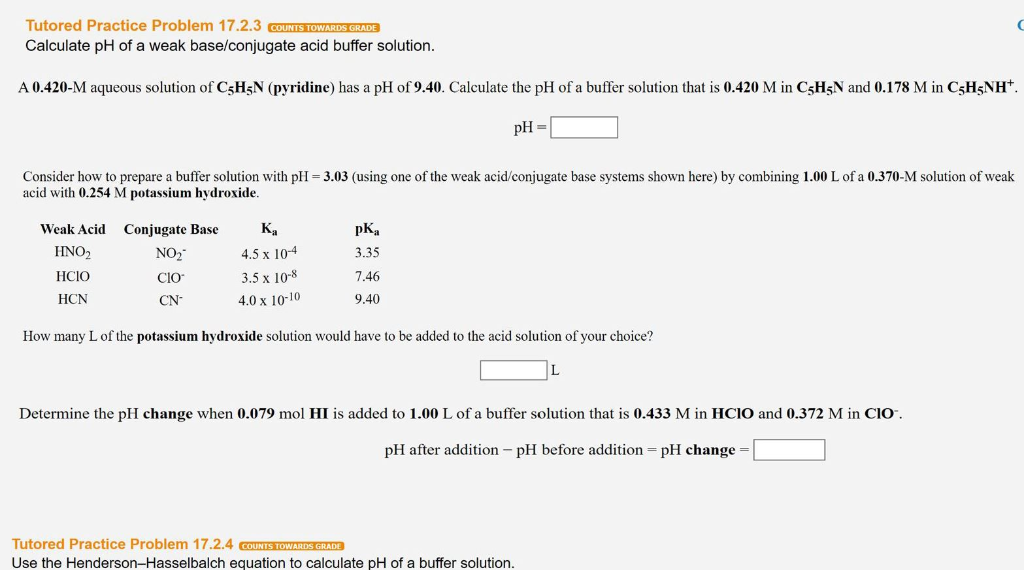Multi part question Tutored Practice Problem 17.2.3 COUNTS TOWARDS GRADE Calculate pH of a weak base/conjugate acid buffer solution A 0.420-M aqueous solution of C5H;N (pyridine) has a pH of 9.40. Calculate the pH of a buffer solution that is 0.420 M in C5H5N and 0.178 M in C;H5NH pH- Consider how to prepare a buffer solution with pH 3.03 (using one of the weak acid/conjugate base systems shown here) by combining 1.00 L of a 0.370-M solution of weak...

• ### Calculate the pH of a 0.0198 M aqueous solution of dimethylamine ((CH),NH, Kn=5.9x104) and the equilibrium concentratio...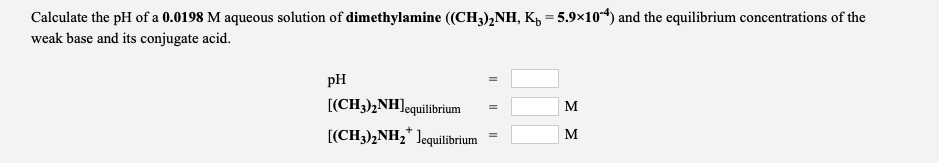Calculate the pH of a 0.0198 M aqueous solution of dimethylamine ((CH),NH, Kn=5.9x104) and the equilibrium concentrations of the weak base and its conjugate acid. PH M [(CH3)2NH)equilibrium [(CH3)2NH2 Lequilibrium = C =